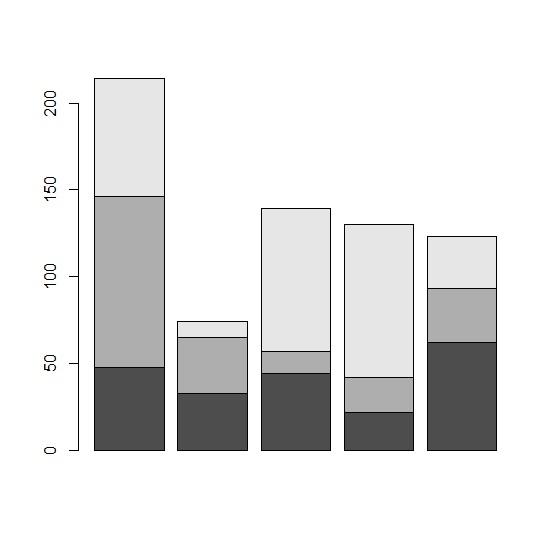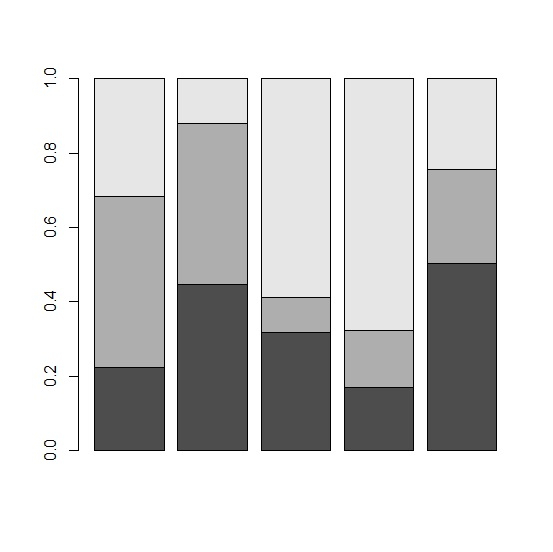# How to create stacked bar plot in which each bar sum to 1 or 100% in R?

A stacked bar plot consists multiple bars in one bar, it shows one category for a categorical variable with its levels. Mostly, the stacked bar chart is created with the count of the levels in each category but if we want to create it with percentage for individual categories of the categorical variables then it can be done as well. We can use prop.table function to create the proportion of levels for each category then create the bar plot.

## Example

Consider the below data frame −

> set.seed(99)
> x1<-sample(1:100,5)
> x2<-sample(1:100,5)
> x3<-sample(1:100,5)
> df<-data.frame(x1,x2,x3)
> df
x1 x2 x3
1 48 98 68
2 33 32 9
3 44 13 82
4 22 20 88
5 62 31 30

Creating a simple stacked bar plot −

> data<-rbind(x1,x2,x3)
> prop.table(data)
[,1] [,2] [,3] [,4] [,5]
x1 0.07058824 0.04852941 0.06470588 0.03235294 0.09117647
x2 0.14411765 0.04705882 0.01911765 0.02941176 0.04558824
x3 0.10000000 0.01323529 0.12058824 0.12941176 0.04411765
> barplot(data)

## OutputCreating a bar plot where each bar sum to 1 −

> barplot(prop.table(data,2))

## Output